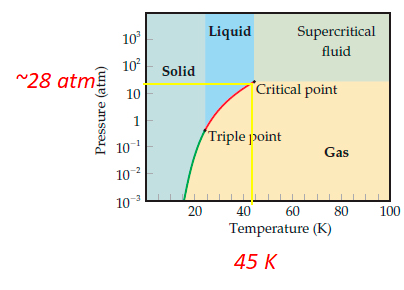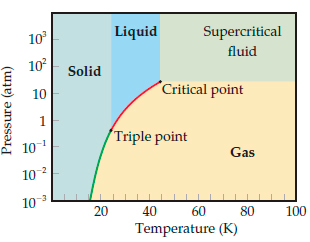# Problem: Use the phase diagram of neon to answer the following questions.What can you say about the strength of the intermolecular forces in neon and argon based on the critical points of Ne and Ar? (critical temperature (Ar) = 150.9  K, critical pressure (Ar) = 48.0  atm)

###### FREE Expert Solution

Recall that a phase diagram shows the transition of matter between solid, liquid, and gas phases as temperature and pressure changes.

The critical point is the pressure and temperature at which the liquid and gas phase are indistinguishable (gas and liquid phase ends)

For Neon, critical point: Tc = ~45 K, Pc = ~28 atm93% (444 ratings)###### Problem Details

Use the phase diagram of neon to answer the following questions.What can you say about the strength of the intermolecular forces in neon and argon based on the critical points of Ne and Ar? (critical temperature (Ar) = 150.9  K, critical pressure (Ar) = 48.0  atm)

Frequently Asked Questions

What scientific concept do you need to know in order to solve this problem?

Our tutors have indicated that to solve this problem you will need to apply the Phase Diagram concept. You can view video lessons to learn Phase Diagram. Or if you need more Phase Diagram practice, you can also practice Phase Diagram practice problems.

What professor is this problem relevant for?

Based on our data, we think this problem is relevant for Professor Bongiorno's class at STATEN ISLAND CUNY.